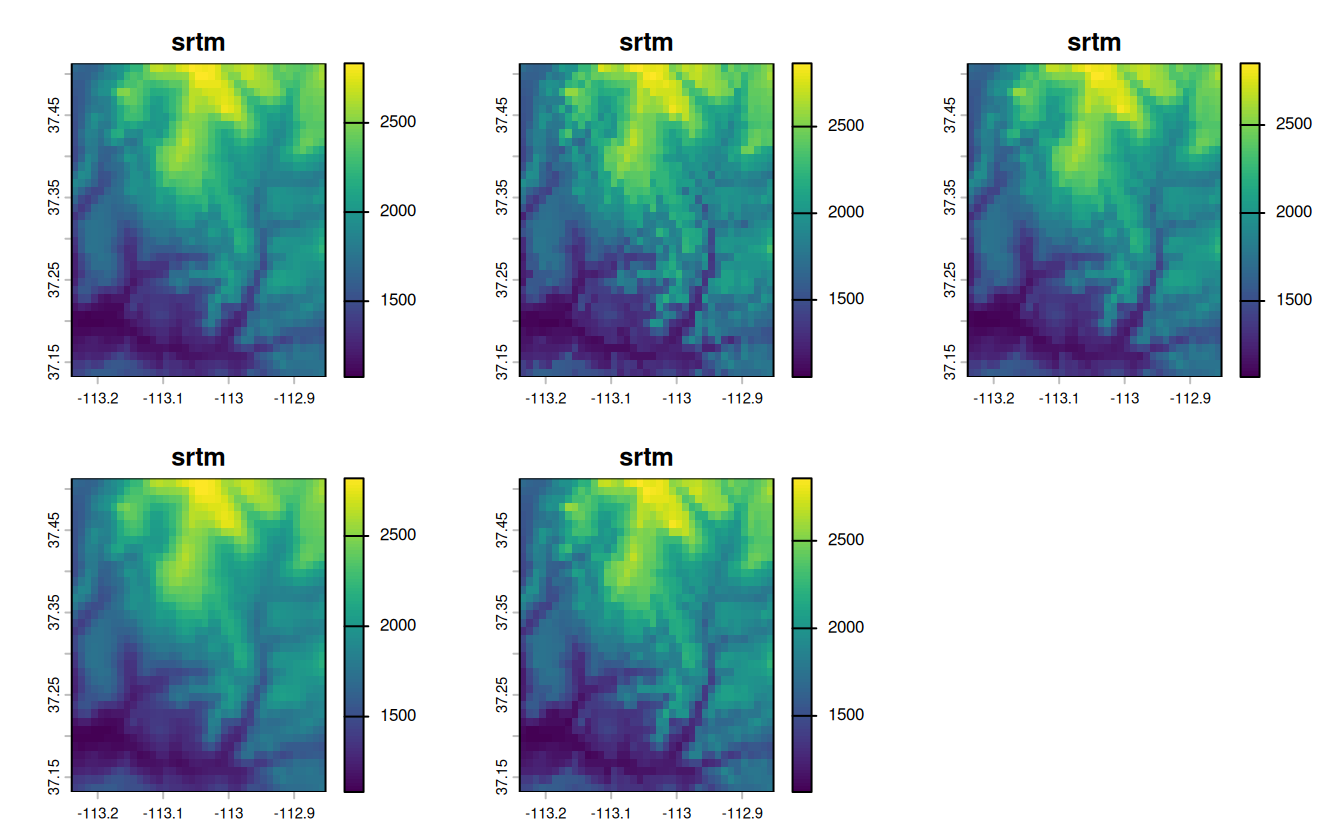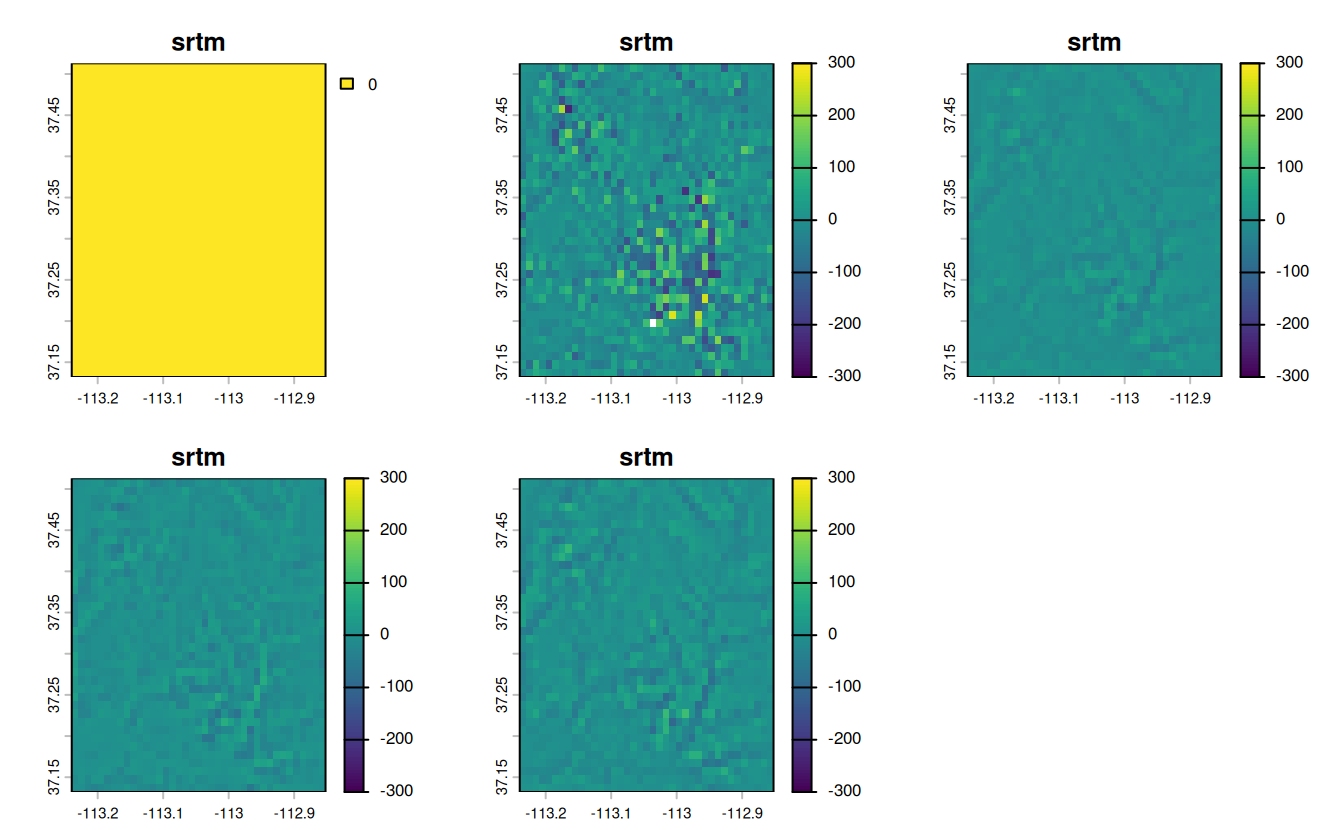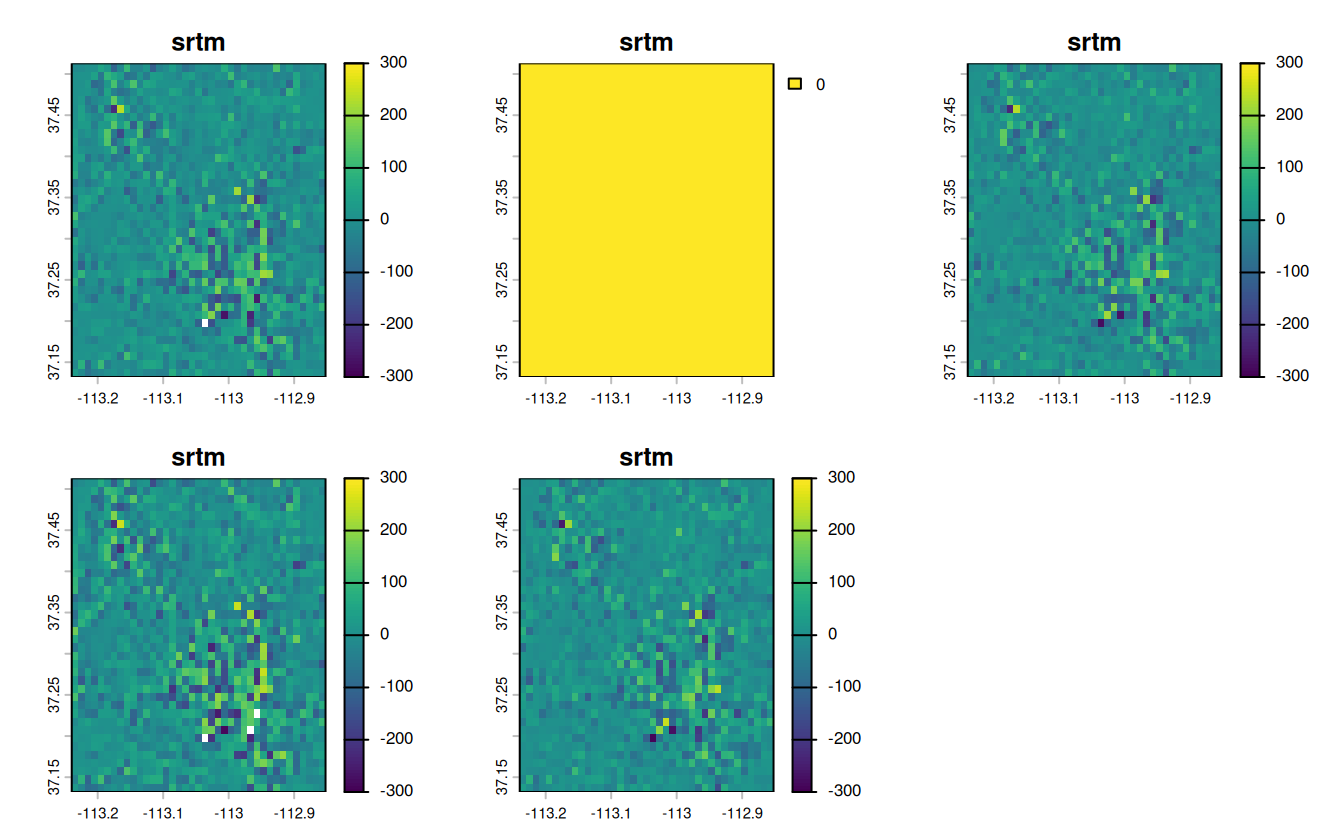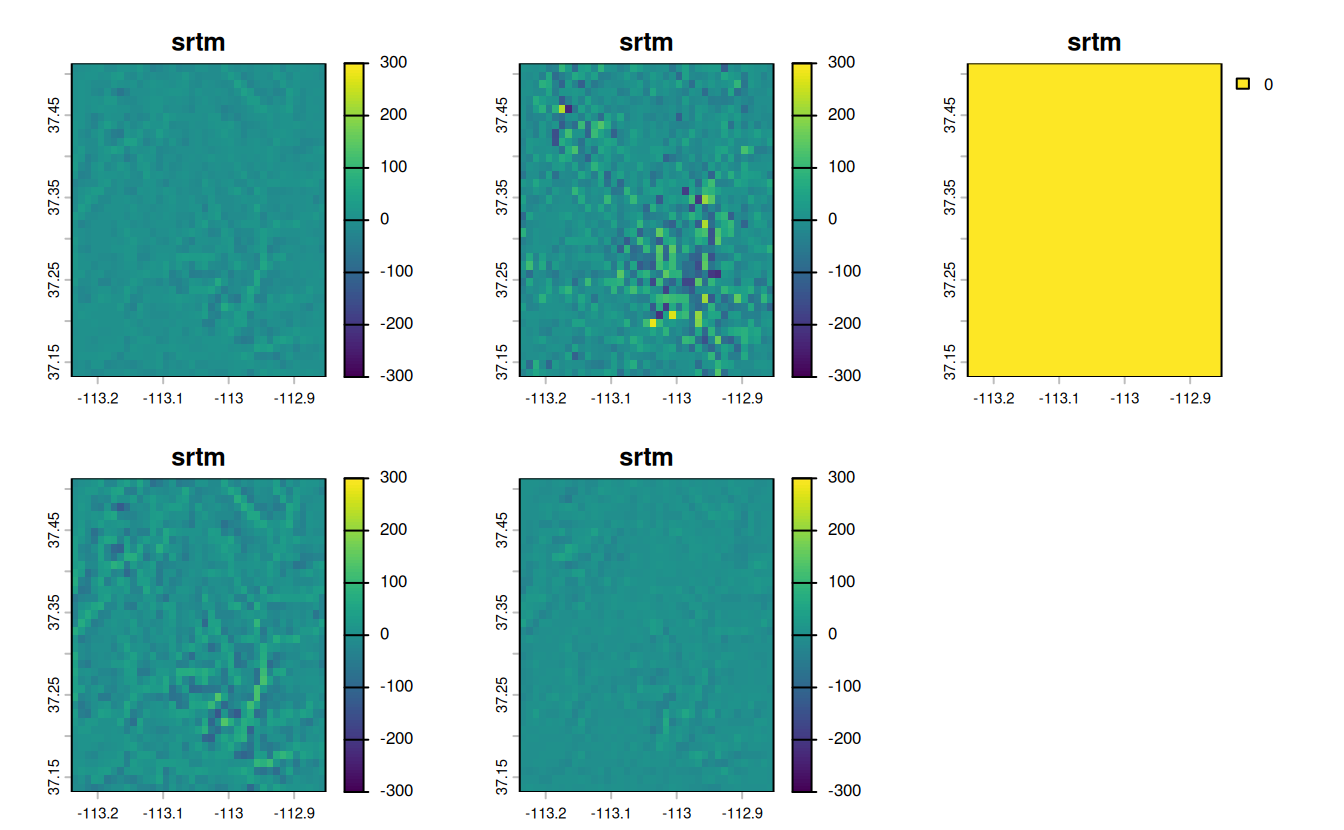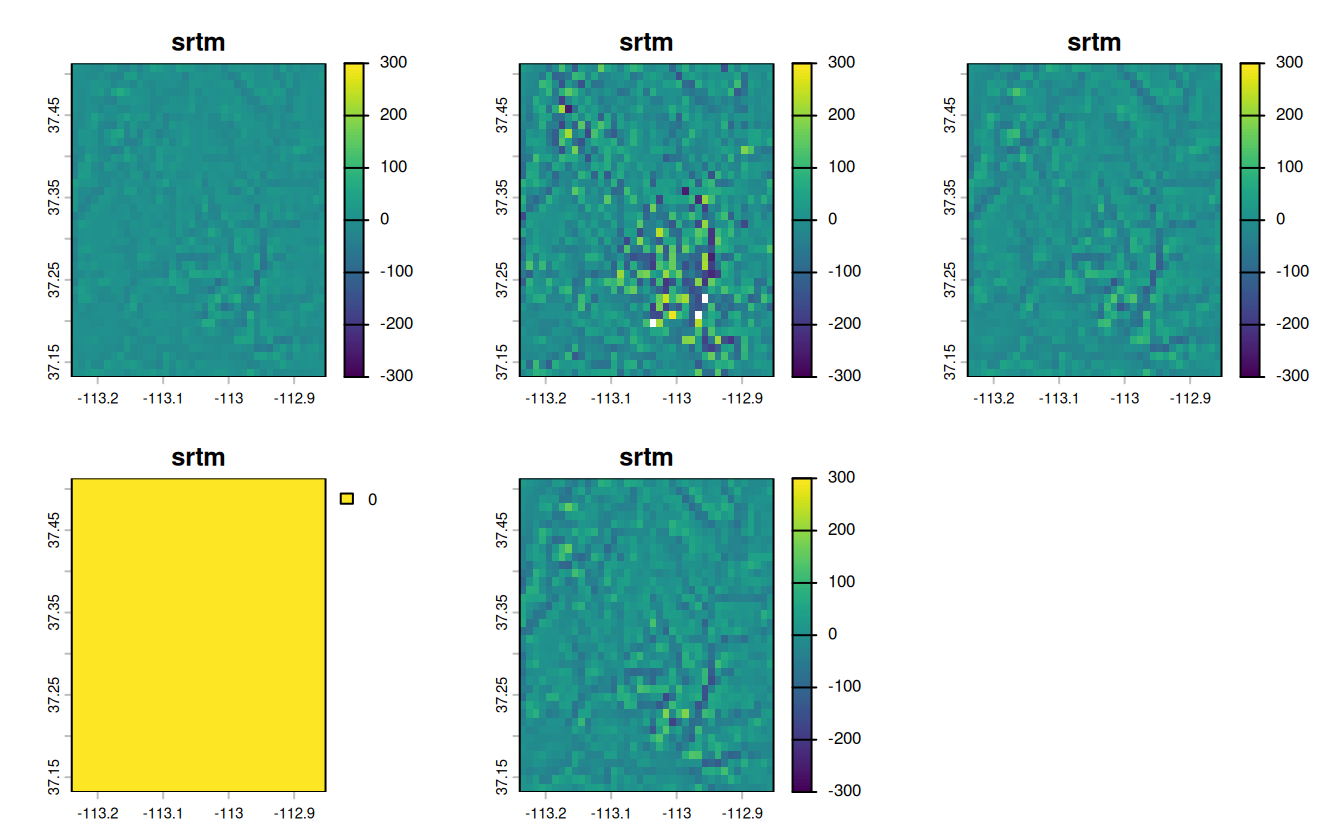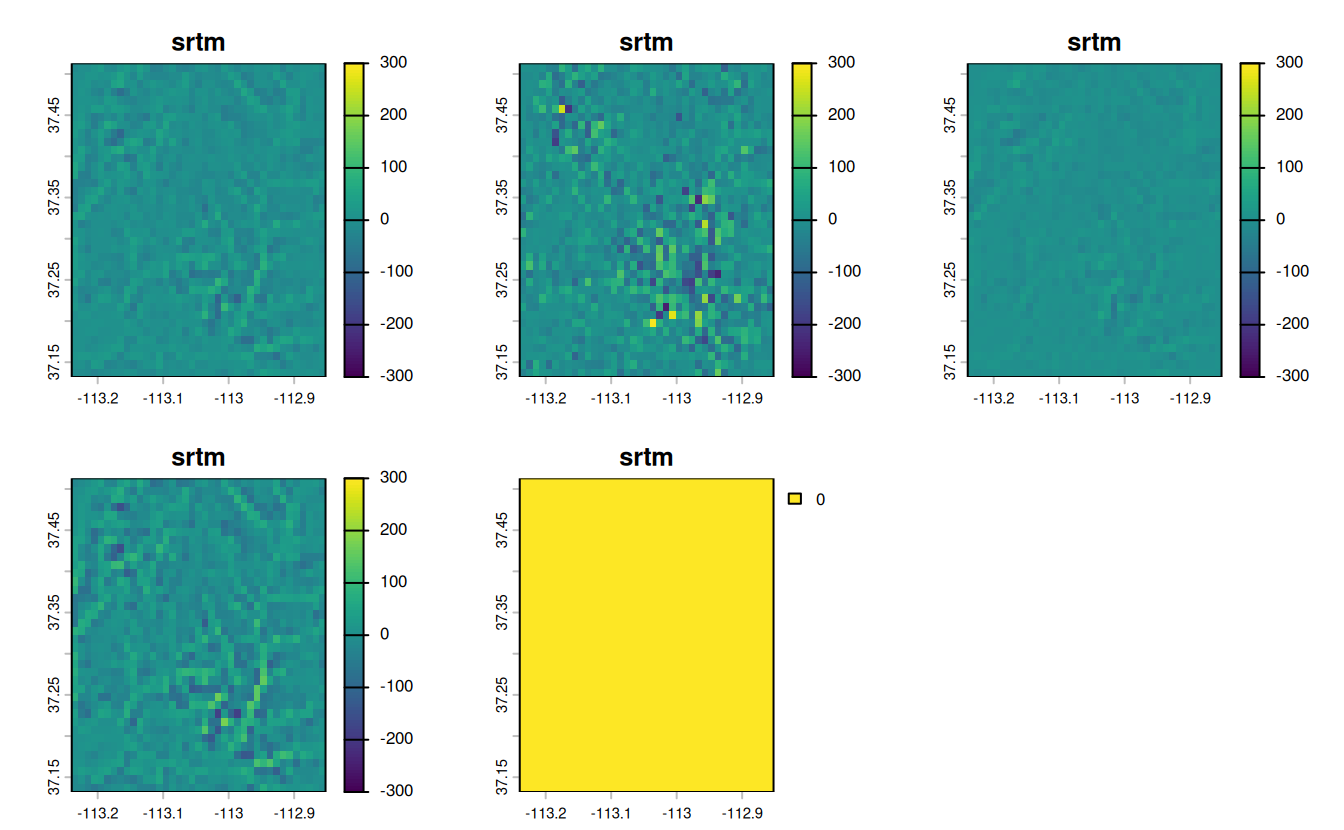# 5 Geometry operations

library(sf)
library(terra)
library(dplyr)
library(spData)
library(spDataLarge)

E1. Generate and plot simplified versions of the nz dataset. Experiment with different values of keep (ranging from 0.5 to 0.00005) for ms_simplify() and dTolerance (from 100 to 100,000) st_simplify().

• At what value does the form of the result start to break down for each method, making New Zealand unrecognizable?
• Advanced: What is different about the geometry type of the results from st_simplify() compared with the geometry type of ms_simplify()? What problems does this create and how can this be resolved?
plot(rmapshaper::ms_simplify(st_geometry(nz), keep = 0.5))
plot(rmapshaper::ms_simplify(st_geometry(nz), keep = 0.05))
# Starts to breakdown here at 0.5% of the points:
plot(rmapshaper::ms_simplify(st_geometry(nz), keep = 0.005))
# At this point no further simplification changes the result
plot(rmapshaper::ms_simplify(st_geometry(nz), keep = 0.0005))
plot(rmapshaper::ms_simplify(st_geometry(nz), keep = 0.00005))
plot(st_simplify(st_geometry(nz), dTolerance = 100))
plot(st_simplify(st_geometry(nz), dTolerance = 1000))
# Starts to breakdown at 10 km:
plot(st_simplify(st_geometry(nz), dTolerance = 10000))
plot(st_simplify(st_geometry(nz), dTolerance = 100000))
plot(st_simplify(st_geometry(nz), dTolerance = 100000, preserveTopology = TRUE))

# Problem: st_simplify returns POLYGON and MULTIPOLYGON results, affecting plotting
# Cast into a single geometry type to resolve this
nz_simple_poly = st_simplify(st_geometry(nz), dTolerance = 10000) |>
st_sfc() |>
st_cast("POLYGON")
#> Warning in st_cast.MULTIPOLYGON(X[[i]], ...): polygon from first part only

#> Warning in st_cast.MULTIPOLYGON(X[[i]], ...): polygon from first part only
nz_simple_multipoly = st_simplify(st_geometry(nz), dTolerance = 10000) |>
st_sfc() |>
st_cast("MULTIPOLYGON")
plot(nz_simple_poly)
length(nz_simple_poly)
#>  16
nrow(nz)
#>  16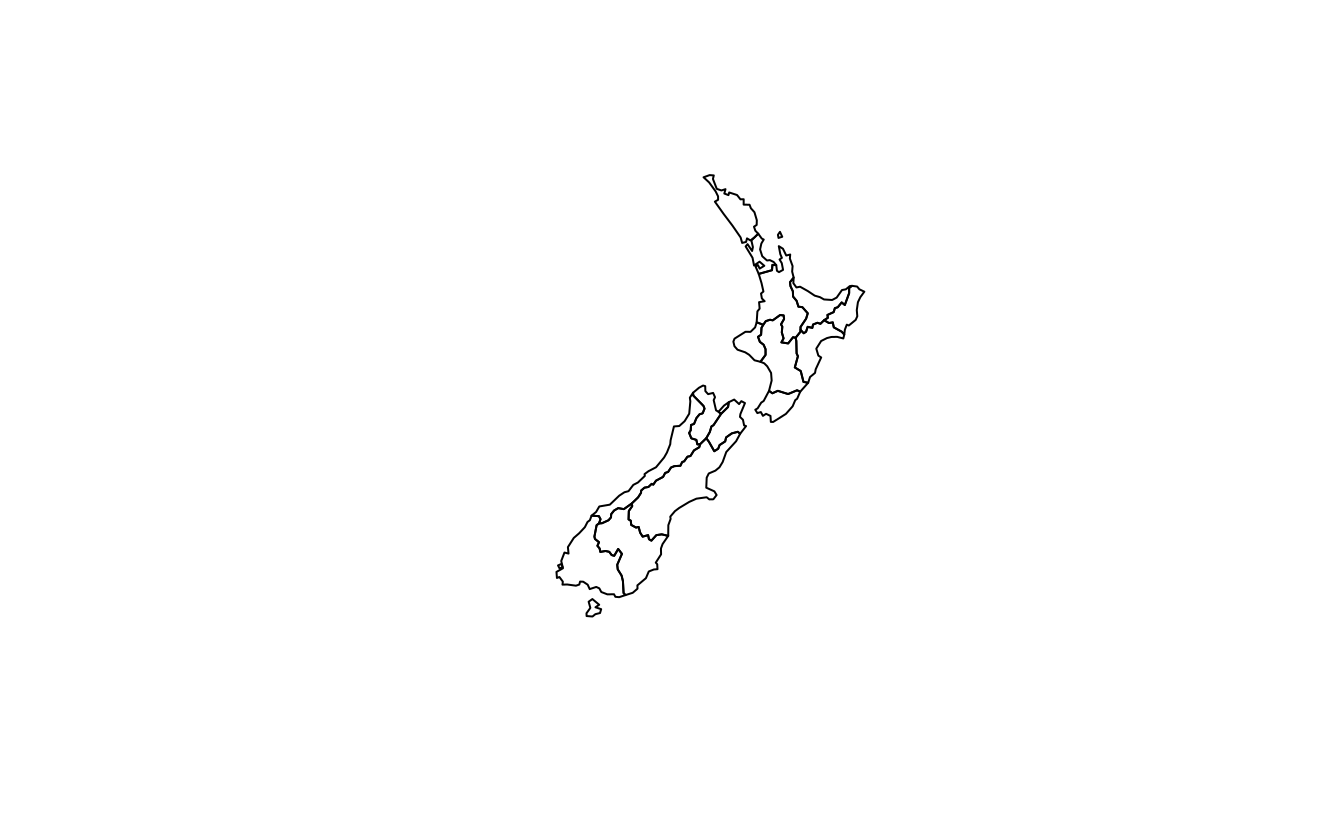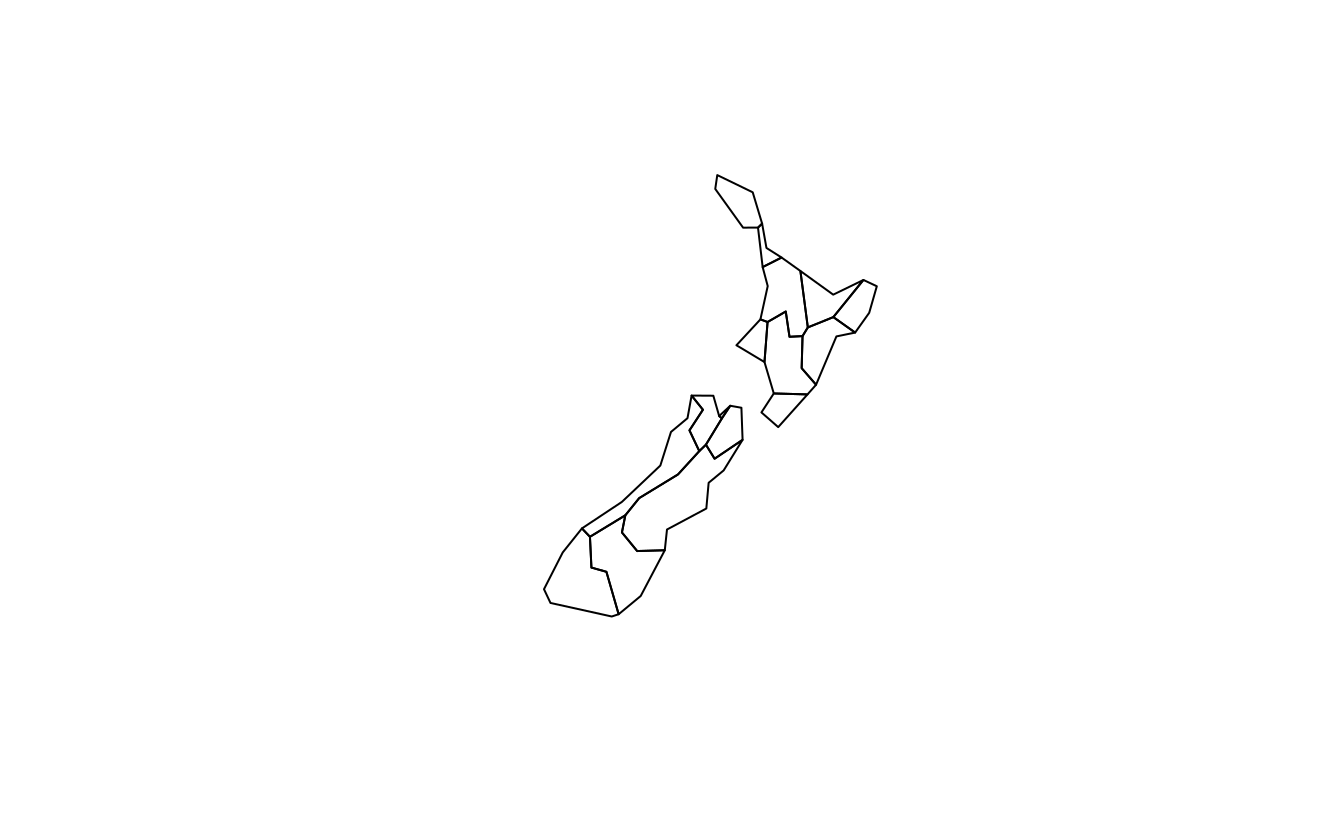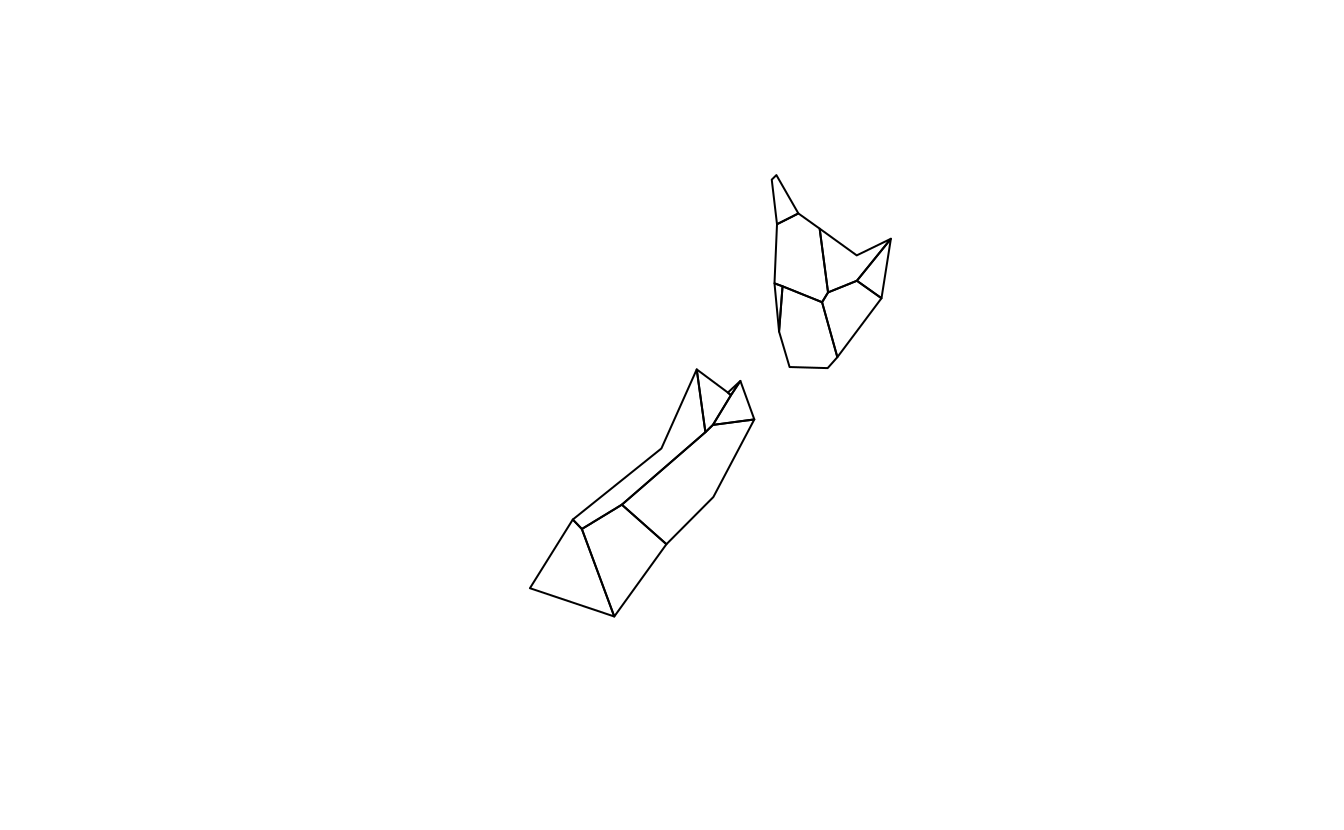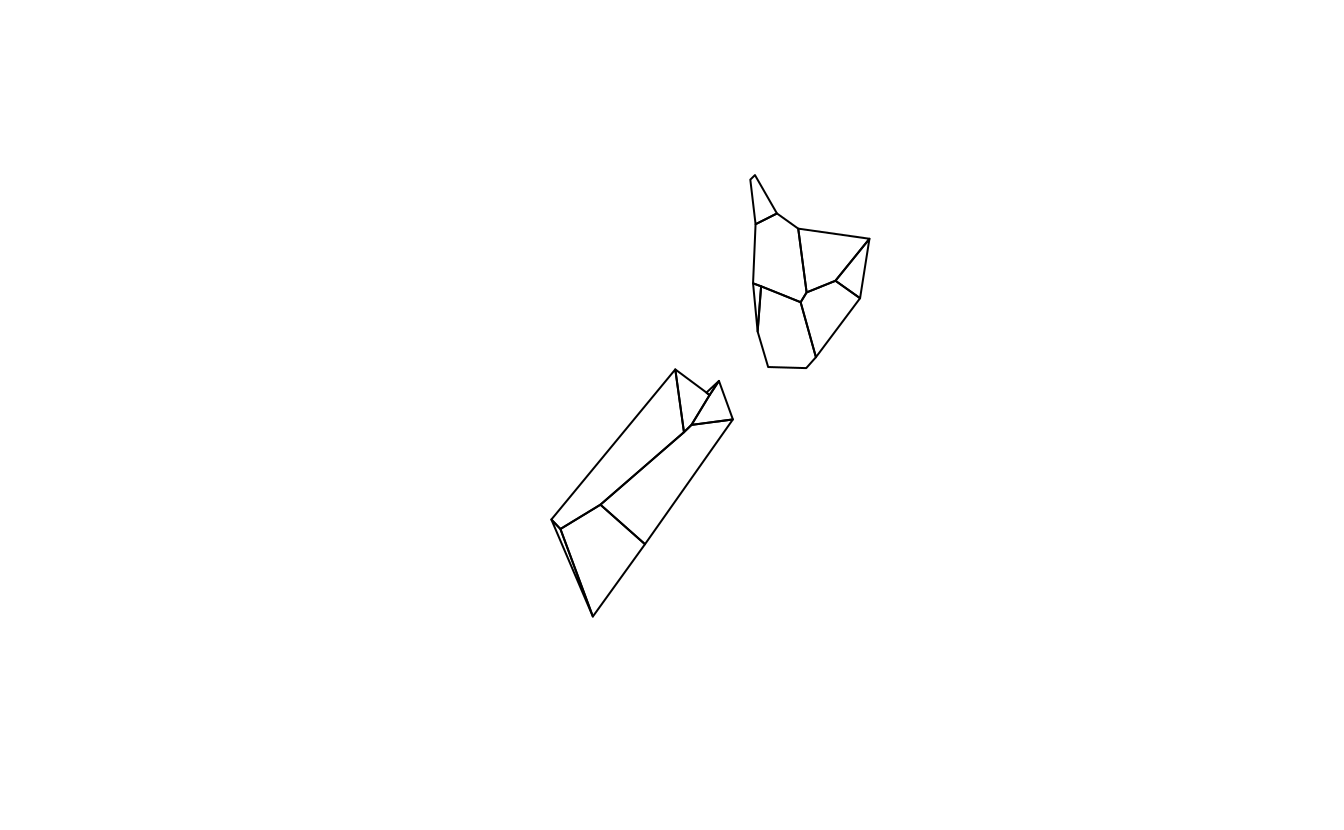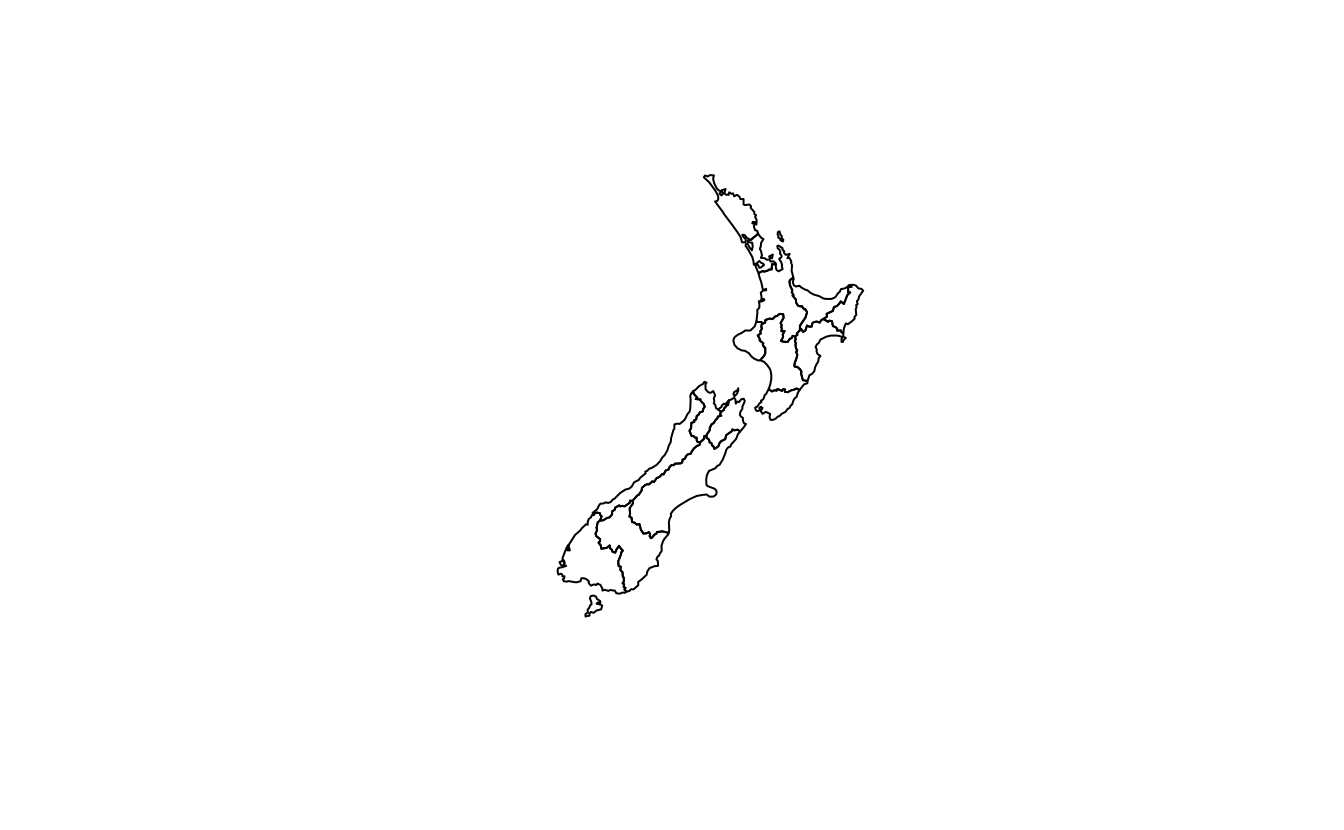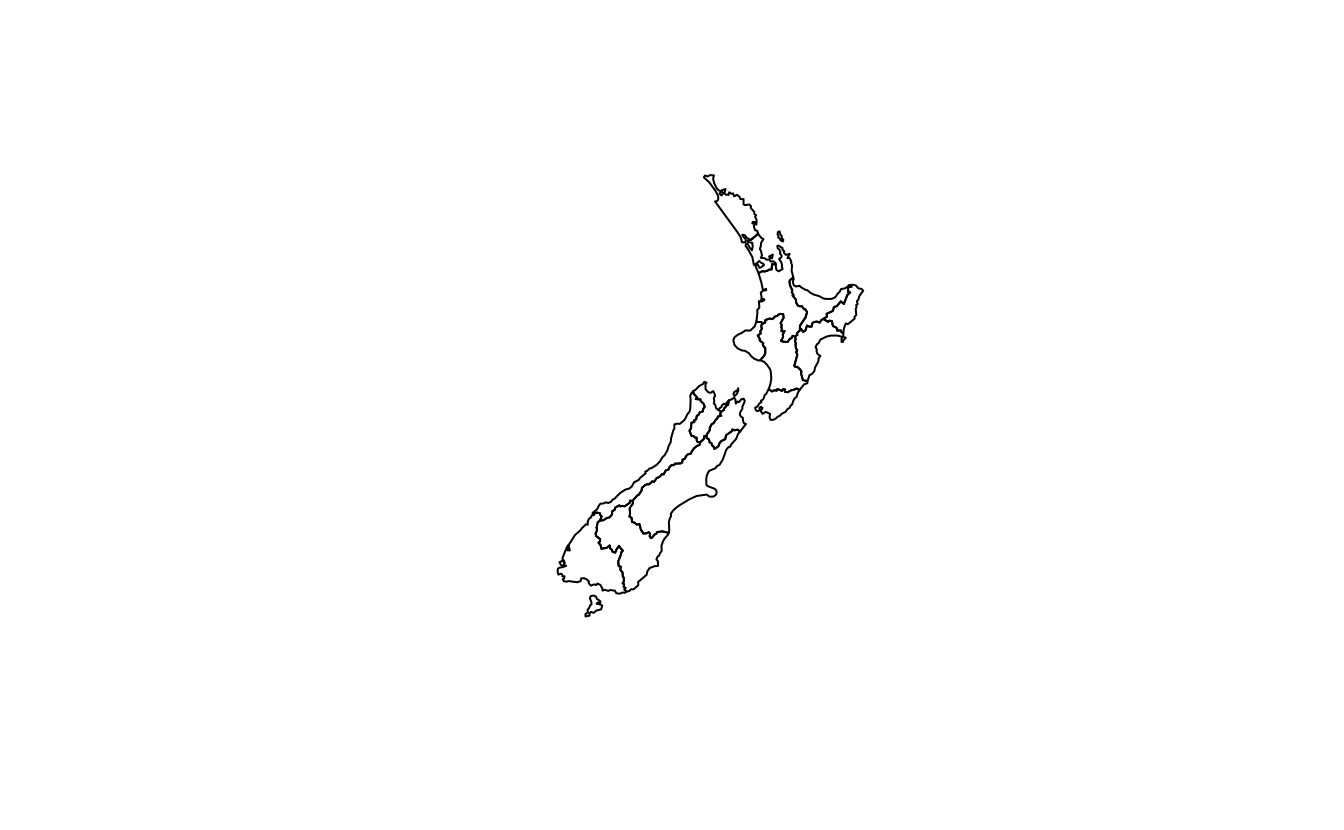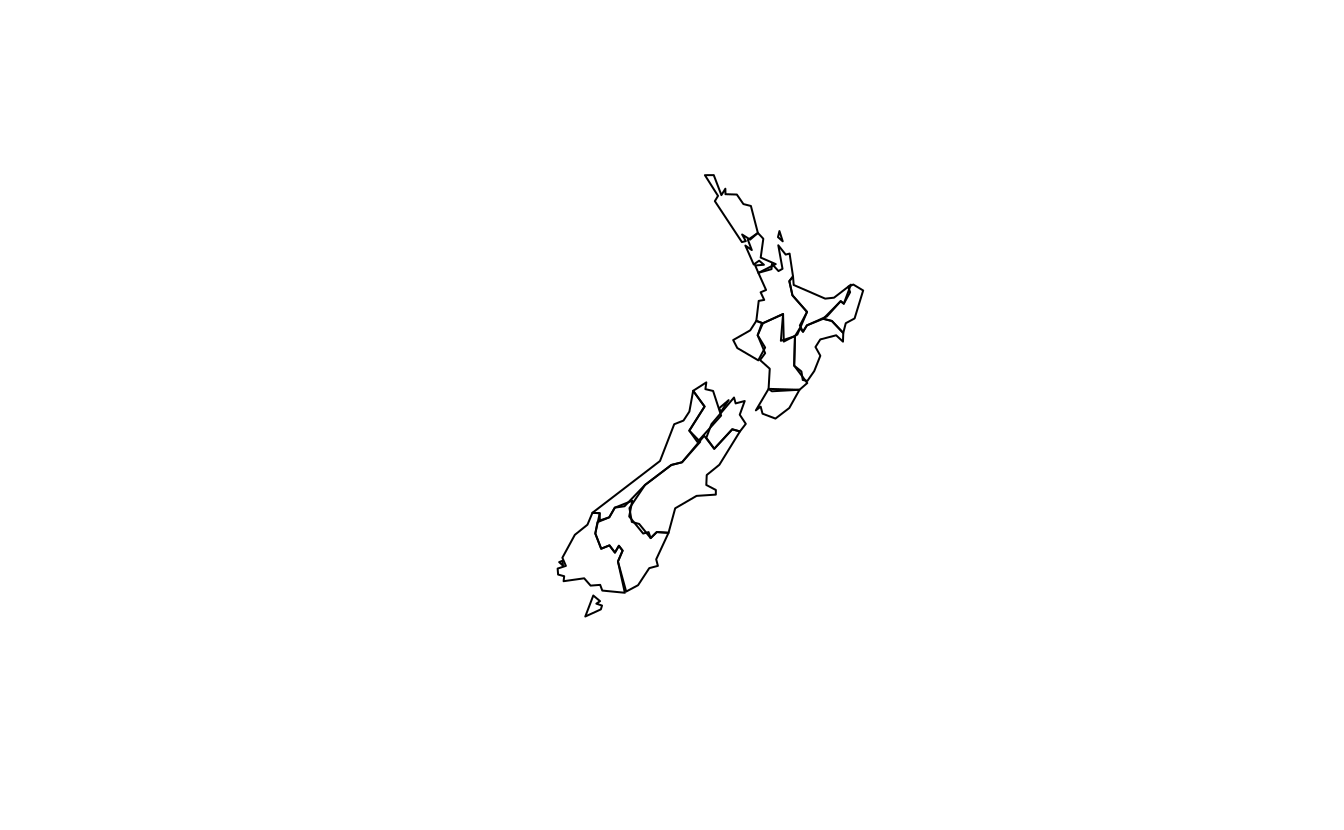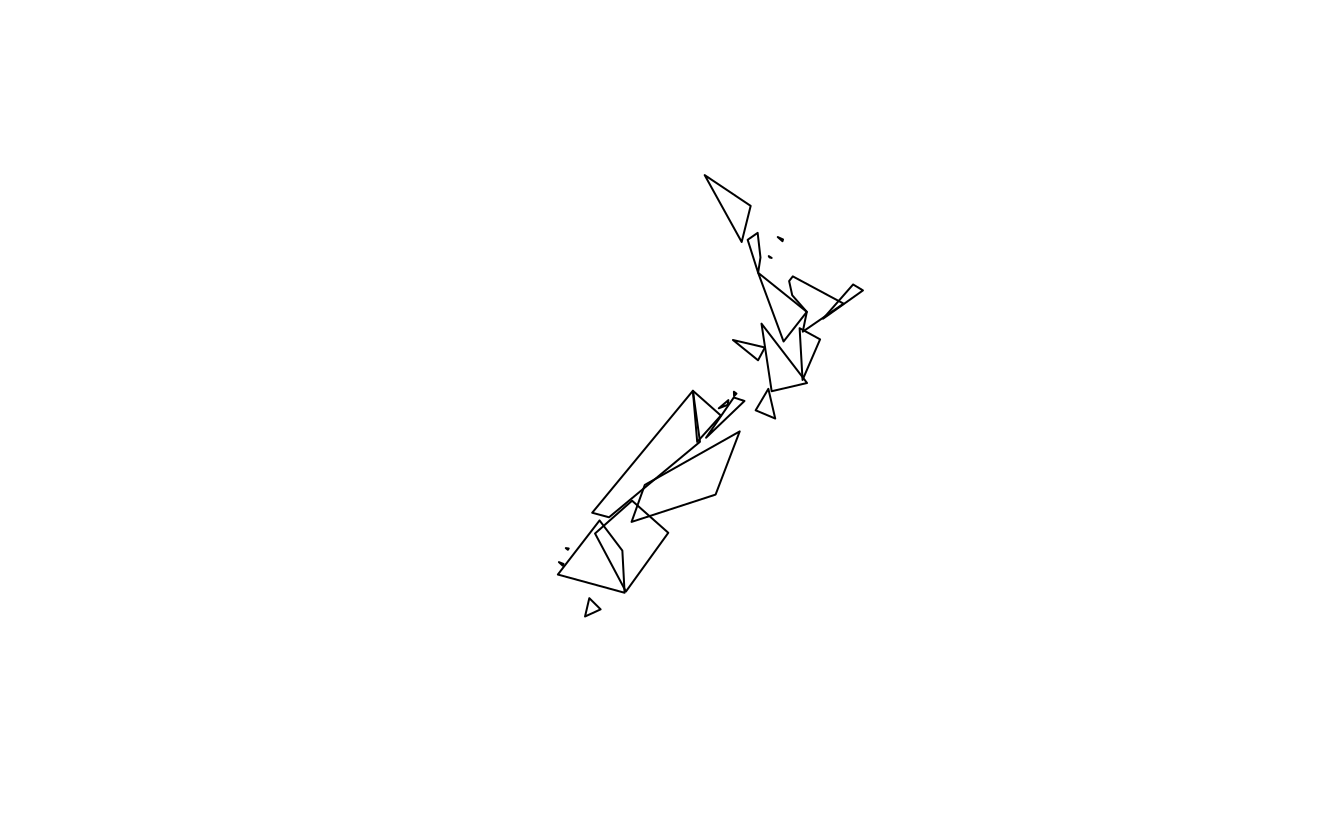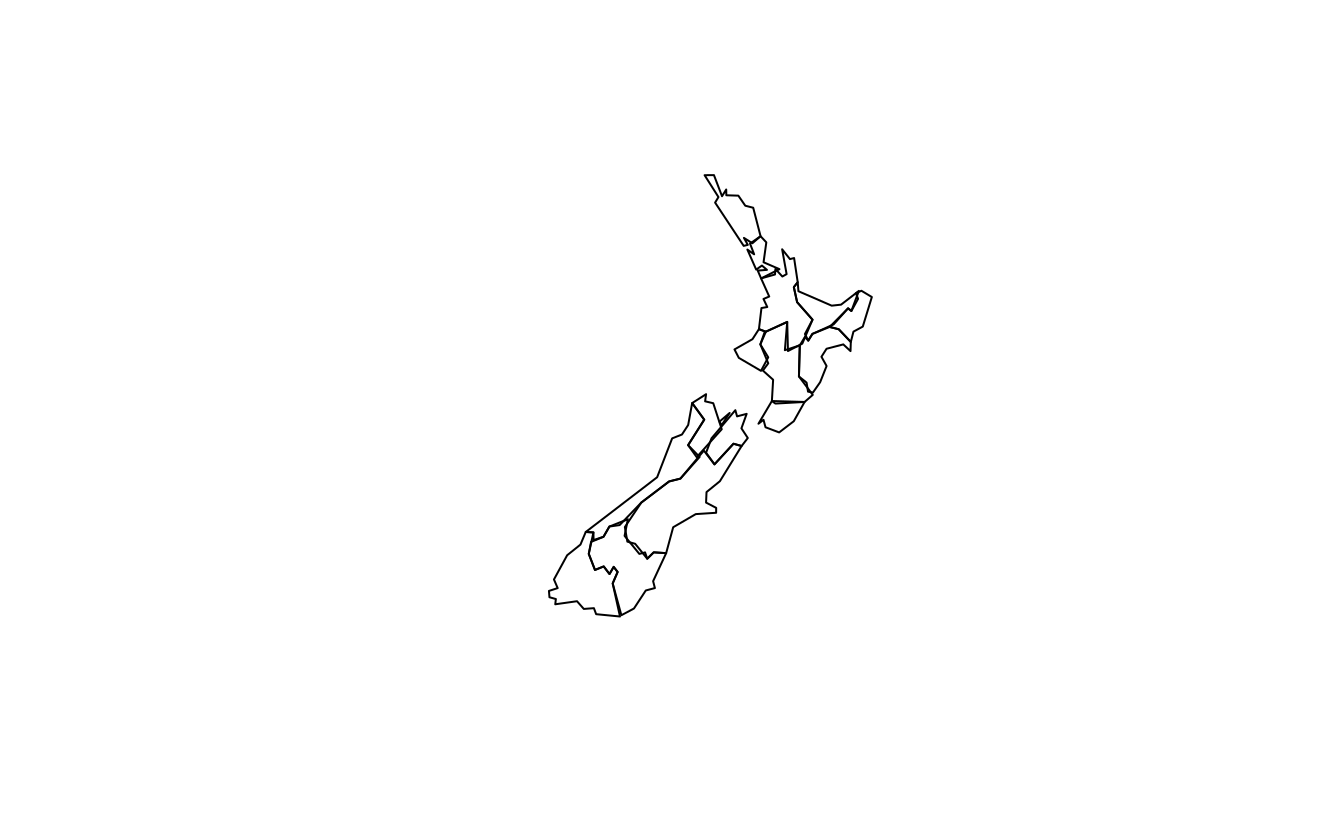E2. In the first exercise in Chapter Spatial data operations it was established that Canterbury region had 70 of the 101 highest points in New Zealand. Using st_buffer(), how many points in nz_height are within 100 km of Canterbury?

canterbury = nz[nz$Name == "Canterbury", ] cant_buff = st_buffer(canterbury, 100) nz_height_near_cant = nz_height[cant_buff, ] nrow(nz_height_near_cant) # 75 - 5 more #>  75 E3. Find the geographic centroid of New Zealand. How far is it from the geographic centroid of Canterbury? cant_cent = st_centroid(canterbury) #> Warning: st_centroid assumes attributes are constant over geometries nz_centre = st_centroid(st_union(nz)) st_distance(cant_cent, nz_centre) # 234 km #> Units: [m] #> [,1] #> [1,] 234193 E4. Most world maps have a north-up orientation. A world map with a south-up orientation could be created by a reflection (one of the affine transformations not mentioned in this chapter) of the world object’s geometry. Write code to do so. Hint: you need to use a two-element vector for this transformation. Bonus: create an upside-down map of your country. world_sfc = st_geometry(world) world_sfc_mirror = world_sfc * c(1, -1) #> Warning in mapply(function(x, y) {: longer argument not a multiple of length of #> shorter plot(world_sfc) plot(world_sfc_mirror) us_states_sfc = st_geometry(us_states) us_states_sfc_mirror = us_states_sfc * c(1, -1) #> Warning in mapply(function(x, y) {: longer argument not a multiple of length of #> shorter plot(us_states_sfc) plot(us_states_sfc_mirror) ## nicer plot # library(ggrepel) # us_states_sfc_mirror_labels = st_centroid(us_states_sfc_mirror) |> # st_coordinates() |> # as_data_frame() |> # mutate(name = us_states$NAME)
# us_states_sfc_mirror_sf = st_set_geometry(us_states, us_states_sfc_mirror)
# ggplot(data = us_states_sfc_mirror_sf) +
#   geom_sf(color = "white") +
#   geom_text_repel(data = us_states_sfc_mirror_labels, mapping = aes(X, Y, label = name), size = 3, min.segment.length = 0) +
#   theme_void()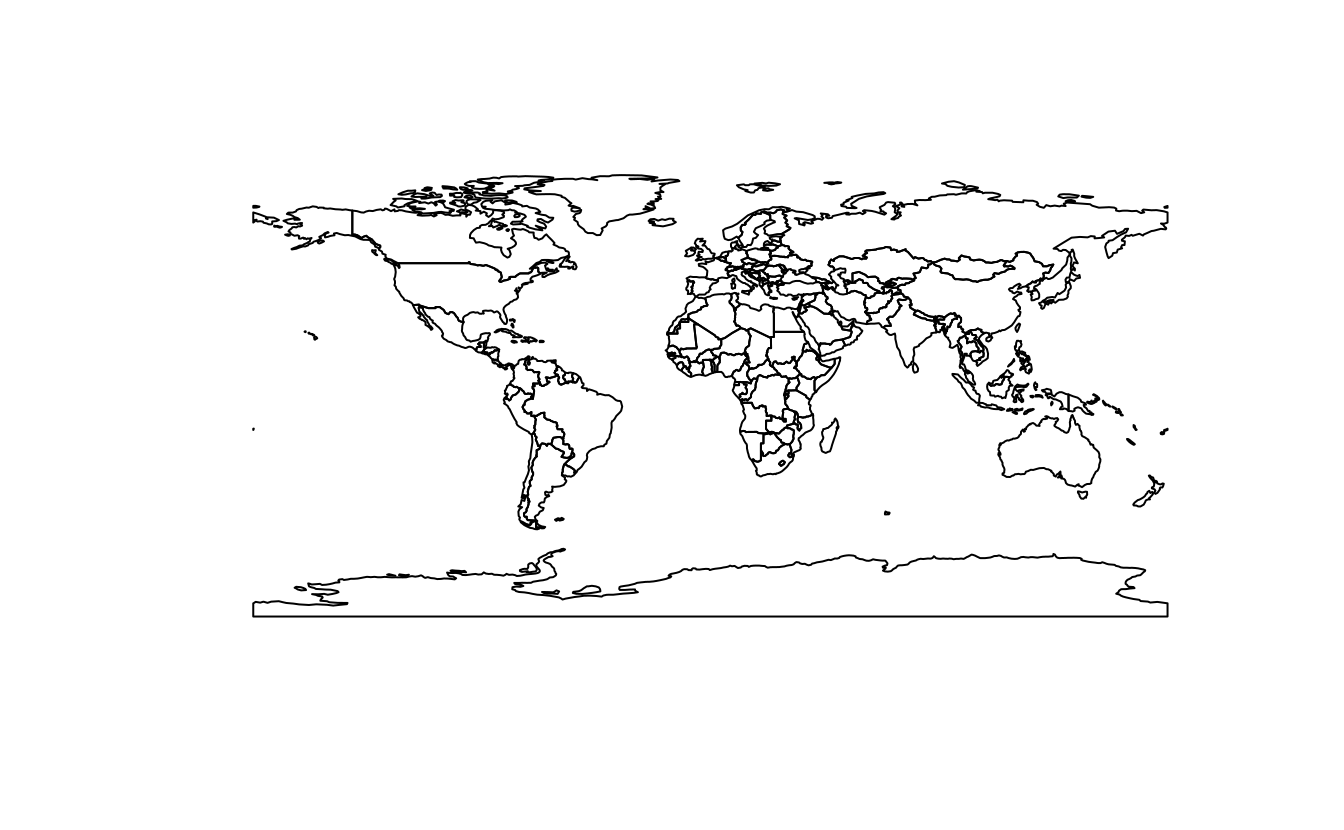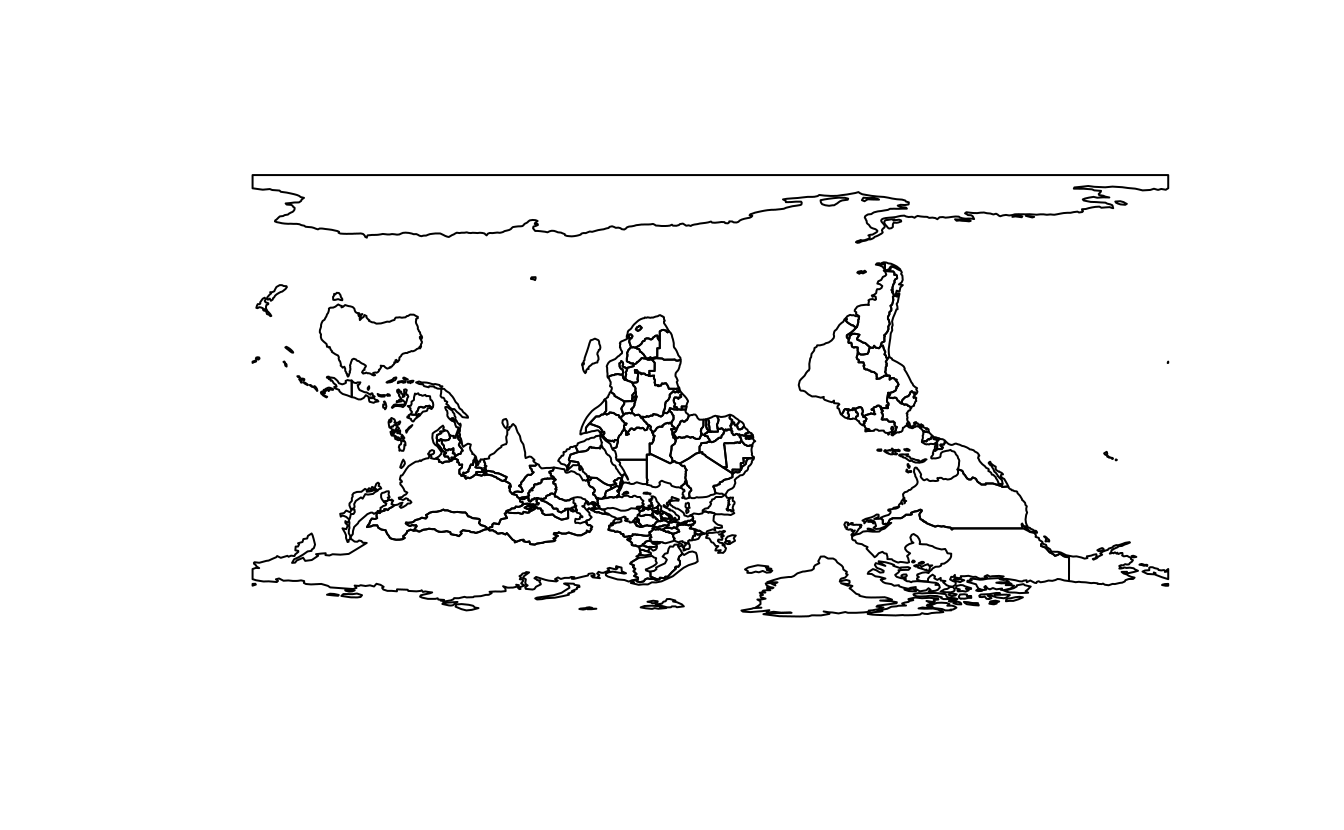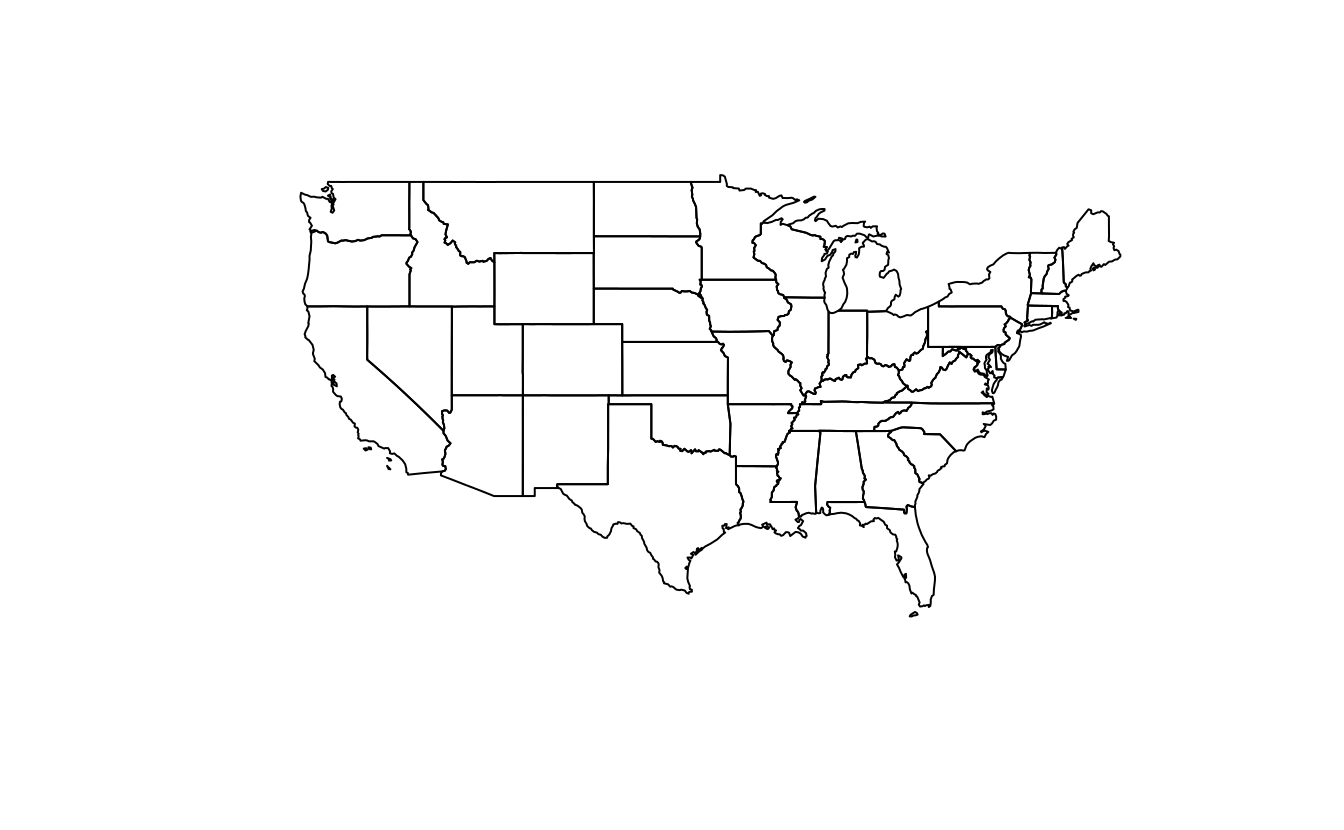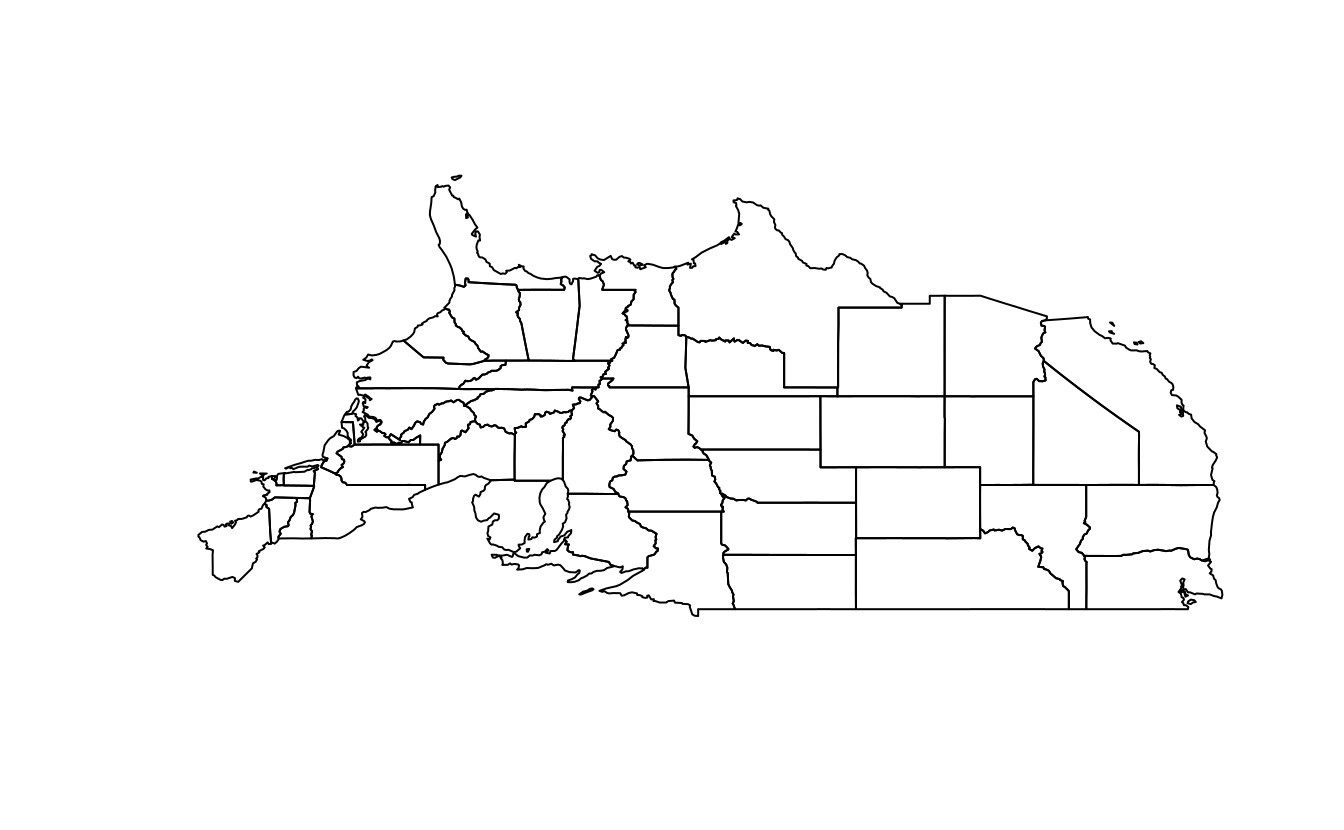E5. Run the code in Section 5.2.6. With reference to the objects created in that section, subset the point in p that is contained within x and y.

• Using base subsetting operators.
• Using an intermediary object created with st_intersection().
p_in_y = p[y]
p_in_xy = p_in_y[x]
x_and_y = st_intersection(x, y)
p[x_and_y]
#> Geometry set for 1 feature
#> Geometry type: POINT
#> Dimension:     XY
#> Bounding box:  xmin: 0.305 ymin: 1.43 xmax: 0.305 ymax: 1.43
#> CRS:           NA
#> POINT (0.305 1.43)

E6. Calculate the length of the boundary lines of US states in meters. Which state has the longest border and which has the shortest? Hint: The st_length function computes the length of a LINESTRING or MULTILINESTRING geometry.

us_states2163 = st_transform(us_states, "EPSG:2163")
us_states_bor = st_cast(us_states2163, "MULTILINESTRING")
us_states_bor\$borders = st_length(us_states_bor)
arrange(us_states_bor, borders)
#> Simple feature collection with 49 features and 7 fields
#> Geometry type: MULTILINESTRING
#> Dimension:     XY
#> Bounding box:  xmin: -2040000 ymin: -2110000 xmax: 2520000 ymax: 731000
#> Projected CRS: NAD27 / US National Atlas Equal Area
#> First 10 features:
#>    GEOID                 NAME   REGION         AREA total_pop_10 total_pop_15
#> 1     11 District of Columbia    South   178 [km^2]       584400       647484
#> 2     44         Rhode Island Norteast  2743 [km^2]      1056389      1053661
#> 3     10             Delaware    South  5182 [km^2]       881278       926454
#> 4     09          Connecticut Norteast 12977 [km^2]      3545837      3593222
#> 5     34           New Jersey Norteast 20274 [km^2]      8721577      8904413
#> 6     50              Vermont Norteast 24866 [km^2]       624258       626604
#> 7     33        New Hampshire Norteast 24026 [km^2]      1313939      1324201
#> 8     25        Massachusetts Norteast 20911 [km^2]      6477096      6705586
#> 9     45       South Carolina    South 80904 [km^2]      4511428      4777576
#> 10    18              Indiana  Midwest 93648 [km^2]      6417398      6568645
#>        borders                       geometry
#> 1    60330 [m] MULTILINESTRING ((1955479 -...
#> 2   304697 [m] MULTILINESTRING ((2338362 4...
#> 3   407939 [m] MULTILINESTRING ((2041302 -...
#> 4   514571 [m] MULTILINESTRING ((2148010 2...
#> 5   747090 [m] MULTILINESTRING ((2062773 -...
#> 6   778660 [m] MULTILINESTRING ((2054045 3...
#> 7   782892 [m] MULTILINESTRING ((2188613 3...
#> 8  1018656 [m] MULTILINESTRING ((2422968 3...
#> 9  1275538 [m] MULTILINESTRING ((1534988 -...
#> 10 1436255 [m] MULTILINESTRING ((1033797 -...
arrange(us_states_bor, -borders)
#> Simple feature collection with 49 features and 7 fields
#> Geometry type: MULTILINESTRING
#> Dimension:     XY
#> Bounding box:  xmin: -2040000 ymin: -2110000 xmax: 2520000 ymax: 731000
#> Projected CRS: NAD27 / US National Atlas Equal Area
#> First 10 features:
#>    GEOID       NAME  REGION          AREA total_pop_10 total_pop_15     borders
#> 1     48      Texas   South 687714 [km^2]     24311891     26538614 4959186 [m]
#> 2     06 California    West 409747 [km^2]     36637290     38421464 3810350 [m]
#> 3     26   Michigan Midwest 151119 [km^2]      9952687      9900571 3579183 [m]
#> 4     12    Florida   South 151052 [km^2]     18511620     19645772 2949048 [m]
#> 5     30    Montana    West 380829 [km^2]       973739      1014699 2826586 [m]
#> 6     16      Idaho    West 216513 [km^2]      1526797      1616547 2570606 [m]
#> 7     27  Minnesota Midwest 218566 [km^2]      5241914      5419171 2567389 [m]
#> 8     51   Virginia   South 105405 [km^2]      7841754      8256630 2407299 [m]
#> 9     35 New Mexico    West 314886 [km^2]      2013122      2084117 2378172 [m]
#> 10    53 Washington    West 175436 [km^2]      6561297      6985464 2344358 [m]
#>                          geometry
#> 1  MULTILINESTRING ((-269579 -...
#> 2  MULTILINESTRING ((-1720565 ...
#> 3  MULTILINESTRING ((1113866 1...
#> 4  MULTILINESTRING ((1855611 -...
#> 5  MULTILINESTRING ((-1165102 ...
#> 6  MULTILINESTRING ((-1298509 ...
#> 7  MULTILINESTRING ((202873 44...
#> 8  MULTILINESTRING ((2104821 -...
#> 9  MULTILINESTRING ((-805019 -...
#> 10 MULTILINESTRING ((-1663690 ...

E7. Read the srtm.tif file into R (srtm = rast(system.file("raster/srtm.tif", package = "spDataLarge"))). This raster has a resolution of 0.00083 by 0.00083 degrees. Change its resolution to 0.01 by 0.01 degrees using all of the method available in the terra package. Visualize the results. Can you notice any differences between the results of these resampling methods?

srtm = rast(system.file("raster/srtm.tif", package = "spDataLarge"))
rast_template = rast(ext(srtm), res = 0.01)
srtm_resampl1 = resample(srtm, y = rast_template, method = "bilinear")
srtm_resampl2 = resample(srtm, y = rast_template, method = "near")
srtm_resampl3 = resample(srtm, y = rast_template, method = "cubic")
srtm_resampl4 = resample(srtm, y = rast_template, method = "cubicspline")
srtm_resampl5 = resample(srtm, y = rast_template, method = "lanczos")

srtm_resampl_all = c(srtm_resampl1, srtm_resampl2, srtm_resampl3,
srtm_resampl4, srtm_resampl5)
plot(srtm_resampl_all)

# differences
plot(srtm_resampl_all - srtm_resampl1, range = c(-300, 300))
plot(srtm_resampl_all - srtm_resampl2, range = c(-300, 300))
plot(srtm_resampl_all - srtm_resampl3, range = c(-300, 300))
plot(srtm_resampl_all - srtm_resampl4, range = c(-300, 300))
plot(srtm_resampl_all - srtm_resampl5, range = c(-300, 300))